# 1.PTA作业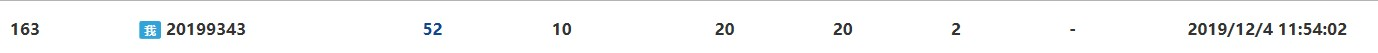## 1.1 6-1 统计某类完全平方数

### 1.1.1 代码截图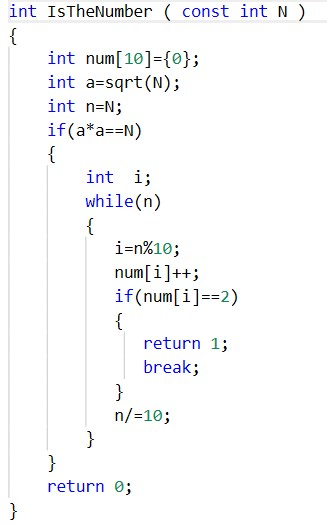### 1.1.2 提交列表及说明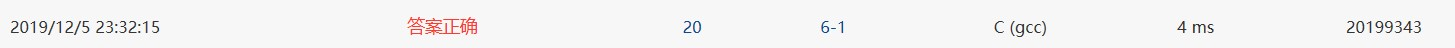### 1.1.3 数据处理

数据表达：定义了num，n,a四个变量，四个变量都是整型变量。



{ 定义 num={0};

{

while(n)
{
i=n%10;
num[i]++;

2)
{

break;
}
n/=10;
}
}

}

### 1.1.4 造测数据

105 500 cnt=6
100 523 cnt=7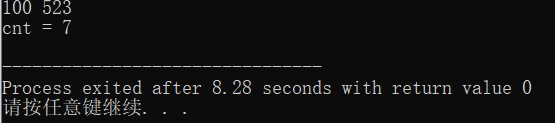## 1.2

int is( int number );
void count_sum( int a, int b );

count = 满足条件的整数个数, sum = 这些整数的和

### 1.2.1 数据处理

{

while(n!=0)
{
t=n除10得余数;
n/=10;
s+=t;
}

}
void count_sum(定义 a, 定义 b )
{

for循环(i=a;i<=b;i++)
{

{
count++;
sum+=i;
}
}
printf("count = %d, sum = %d\n",count,sum);
}

### 1.2.2 提交列表与说明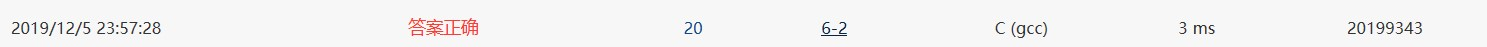### 1.2.3 代码截图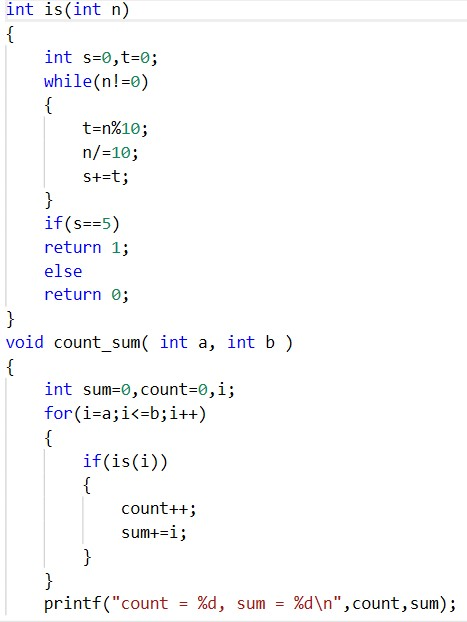### 1.2.4 造测试数据

104 999 count = 15, sum = 3720
100 1034 count =19，sum =7790

# 2.代码互评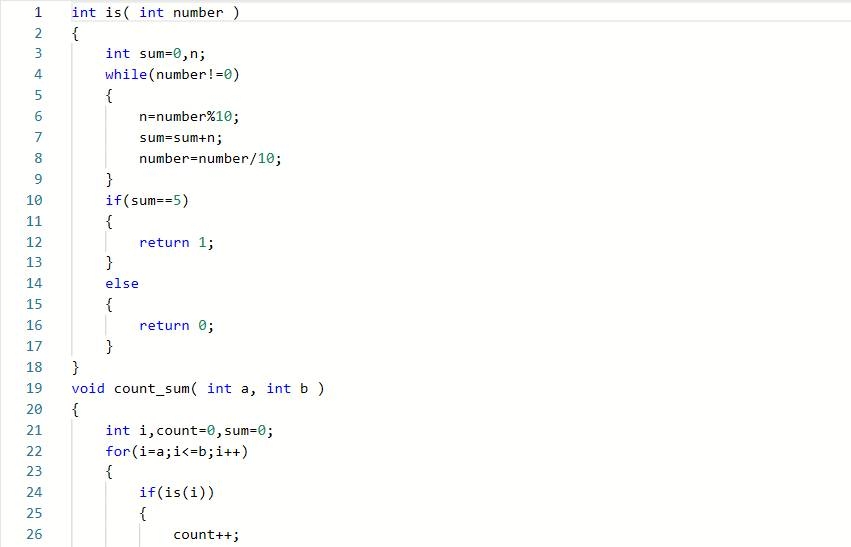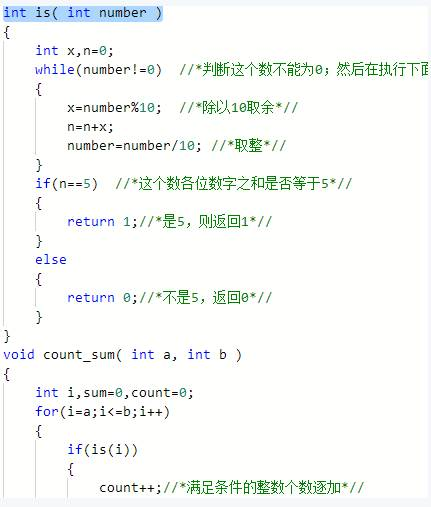# 3.学习总结

## 3.2 累计代码行与博客字数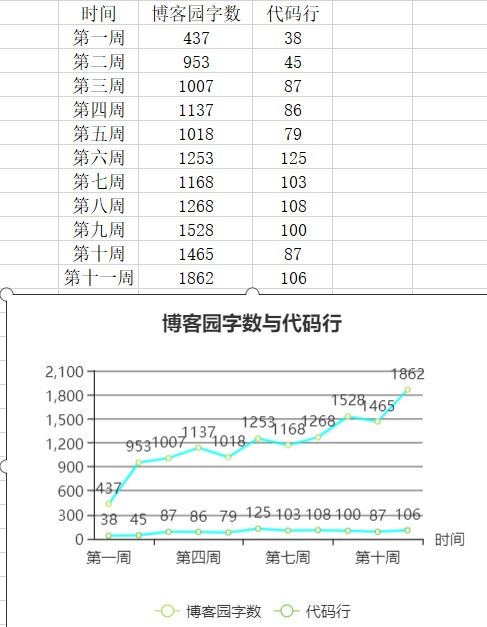## 3.3 本周学习总结和感悟

### 3.3.1 学习内容总结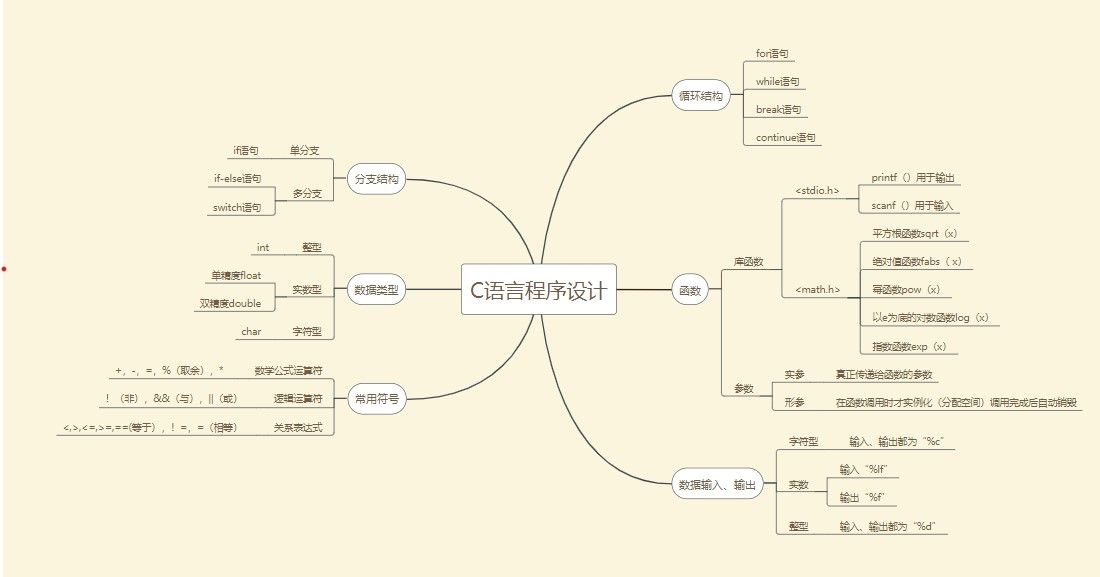### 3.3.2 学习体会

1.本周主要学习了函数，相比以前所学的循环而言函数更加的复杂，要考虑许多东西还要考虑我们所设置的参数属于某种参数。
2.函数中的参数主要分为局部参数、全局参数，在编写时要考虑你所设置的参数的存活时间，这个部分是函数的一个难点，需要花时间去搞懂。
3.这一周的函数在听完之后是雨里雾里的，觉得有些些懂了，但是到了实际操作时又觉得啥也不不会了。

posted on 2019-12-06 19:39  王岐桐  阅读(149)  评论(2编辑  收藏